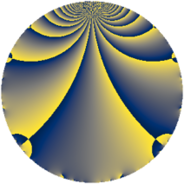# Properties

 Label 825.2.aLevel $825$ Weight $2$ Character orbit 825.a Rep. character $\chi_{825}(1,\cdot)$ Character field $\Q$ Dimension $32$ Newform subspaces $14$ Sturm bound $240$ Trace bound $7$

# Related objects

## Defining parameters

 Level: $$N$$ $$=$$ $$825 = 3 \cdot 5^{2} \cdot 11$$ Weight: $$k$$ $$=$$ $$2$$ Character orbit: $$[\chi]$$ $$=$$ 825.a (trivial) Character field: $$\Q$$ Newform subspaces: $$14$$ Sturm bound: $$240$$ Trace bound: $$7$$ Distinguishing $$T_p$$: $$2$$, $$7$$

## Dimensions

The following table gives the dimensions of various subspaces of $$M_{2}(\Gamma_0(825))$$.

Total New Old
Modular forms 132 32 100
Cusp forms 109 32 77
Eisenstein series 23 0 23

The following table gives the dimensions of the cuspidal new subspaces with specified eigenvalues for the Atkin-Lehner operators and the Fricke involution.

$$3$$$$5$$$$11$$FrickeDim.
$$+$$$$+$$$$+$$$$+$$$$3$$
$$+$$$$+$$$$-$$$$-$$$$6$$
$$+$$$$-$$$$+$$$$-$$$$5$$
$$+$$$$-$$$$-$$$$+$$$$3$$
$$-$$$$+$$$$+$$$$-$$$$4$$
$$-$$$$+$$$$-$$$$+$$$$1$$
$$-$$$$-$$$$+$$$$+$$$$4$$
$$-$$$$-$$$$-$$$$-$$$$6$$
Plus space$$+$$$$11$$
Minus space$$-$$$$21$$

## Trace form

 $$32q + 2q^{2} - 2q^{3} + 36q^{4} + 4q^{6} - 4q^{7} + 18q^{8} + 32q^{9} + O(q^{10})$$ $$32q + 2q^{2} - 2q^{3} + 36q^{4} + 4q^{6} - 4q^{7} + 18q^{8} + 32q^{9} - 6q^{12} + 20q^{14} + 52q^{16} + 12q^{17} + 2q^{18} - 8q^{19} - 16q^{21} - 2q^{22} + 12q^{24} - 44q^{26} - 2q^{27} - 4q^{28} - 12q^{29} + 12q^{31} + 18q^{32} - 2q^{33} + 8q^{34} + 36q^{36} - 16q^{37} - 4q^{38} - 16q^{39} - 20q^{41} + 12q^{42} + 4q^{43} + 8q^{44} - 8q^{46} + 8q^{47} + 2q^{48} + 12q^{49} + 56q^{52} + 16q^{53} + 4q^{54} + 36q^{56} - 20q^{57} + 32q^{59} - 20q^{61} - 32q^{62} - 4q^{63} + 76q^{64} + 4q^{66} - 8q^{67} - 52q^{68} + 16q^{69} + 24q^{71} + 18q^{72} + 24q^{73} + 4q^{74} - 20q^{76} - 4q^{77} + 16q^{78} - 4q^{79} + 32q^{81} - 16q^{83} - 36q^{84} + 20q^{86} + 6q^{88} + 16q^{89} - 4q^{91} - 8q^{93} - 64q^{94} - 12q^{96} - 16q^{97} - 70q^{98} + O(q^{100})$$

## Decomposition of $$S_{2}^{\mathrm{new}}(\Gamma_0(825))$$ into newform subspaces

Label Dim. $$A$$ Field CM Traces A-L signs $q$-expansion
$$a_2$$ $$a_3$$ $$a_5$$ $$a_7$$ 3 5 11
825.2.a.a $$1$$ $$6.588$$ $$\Q$$ None $$-1$$ $$1$$ $$0$$ $$-4$$ $$-$$ $$+$$ $$-$$ $$q-q^{2}+q^{3}-q^{4}-q^{6}-4q^{7}+3q^{8}+\cdots$$
825.2.a.b $$1$$ $$6.588$$ $$\Q$$ None $$0$$ $$-1$$ $$0$$ $$1$$ $$+$$ $$+$$ $$+$$ $$q-q^{3}-2q^{4}+q^{7}+q^{9}-q^{11}+2q^{12}+\cdots$$
825.2.a.c $$1$$ $$6.588$$ $$\Q$$ None $$0$$ $$1$$ $$0$$ $$-1$$ $$-$$ $$-$$ $$+$$ $$q+q^{3}-2q^{4}-q^{7}+q^{9}-q^{11}-2q^{12}+\cdots$$
825.2.a.d $$2$$ $$6.588$$ $$\Q(\sqrt{2})$$ None $$-2$$ $$-2$$ $$0$$ $$2$$ $$+$$ $$-$$ $$+$$ $$q+(-1+\beta )q^{2}-q^{3}+(1-2\beta )q^{4}+(1+\cdots)q^{6}+\cdots$$
825.2.a.e $$2$$ $$6.588$$ $$\Q(\sqrt{3})$$ None $$0$$ $$-2$$ $$0$$ $$-4$$ $$+$$ $$+$$ $$+$$ $$q+\beta q^{2}-q^{3}+q^{4}-\beta q^{6}-2q^{7}-\beta q^{8}+\cdots$$
825.2.a.f $$2$$ $$6.588$$ $$\Q(\sqrt{2})$$ None $$2$$ $$2$$ $$0$$ $$-2$$ $$-$$ $$+$$ $$+$$ $$q+(1+\beta )q^{2}+q^{3}+(1+2\beta )q^{4}+(1+\beta )q^{6}+\cdots$$
825.2.a.g $$2$$ $$6.588$$ $$\Q(\sqrt{2})$$ None $$2$$ $$2$$ $$0$$ $$4$$ $$-$$ $$+$$ $$+$$ $$q+(1+\beta )q^{2}+q^{3}+(1+2\beta )q^{4}+(1+\beta )q^{6}+\cdots$$
825.2.a.h $$3$$ $$6.588$$ 3.3.148.1 None $$-3$$ $$3$$ $$0$$ $$-8$$ $$-$$ $$-$$ $$+$$ $$q+(-1-\beta _{2})q^{2}+q^{3}+(2-\beta _{1}+\beta _{2})q^{4}+\cdots$$
825.2.a.i $$3$$ $$6.588$$ 3.3.568.1 None $$-2$$ $$-3$$ $$0$$ $$3$$ $$+$$ $$+$$ $$-$$ $$q+(-1+\beta _{1})q^{2}-q^{3}+(3+\beta _{2})q^{4}+\cdots$$
825.2.a.j $$3$$ $$6.588$$ 3.3.148.1 None $$-1$$ $$-3$$ $$0$$ $$-4$$ $$+$$ $$-$$ $$-$$ $$q-\beta _{1}q^{2}-q^{3}+(\beta _{1}+\beta _{2})q^{4}+\beta _{1}q^{6}+\cdots$$
825.2.a.k $$3$$ $$6.588$$ 3.3.148.1 None $$1$$ $$-3$$ $$0$$ $$0$$ $$+$$ $$+$$ $$-$$ $$q+\beta _{1}q^{2}-q^{3}+(1+\beta _{1}+\beta _{2})q^{4}-\beta _{1}q^{6}+\cdots$$
825.2.a.l $$3$$ $$6.588$$ 3.3.148.1 None $$1$$ $$3$$ $$0$$ $$4$$ $$-$$ $$-$$ $$-$$ $$q+\beta _{1}q^{2}+q^{3}+(\beta _{1}+\beta _{2})q^{4}+\beta _{1}q^{6}+\cdots$$
825.2.a.m $$3$$ $$6.588$$ 3.3.568.1 None $$2$$ $$3$$ $$0$$ $$-3$$ $$-$$ $$-$$ $$-$$ $$q+(1-\beta _{1})q^{2}+q^{3}+(3+\beta _{2})q^{4}+(1+\cdots)q^{6}+\cdots$$
825.2.a.n $$3$$ $$6.588$$ 3.3.148.1 None $$3$$ $$-3$$ $$0$$ $$8$$ $$+$$ $$-$$ $$+$$ $$q+(1+\beta _{2})q^{2}-q^{3}+(2-\beta _{1}+\beta _{2})q^{4}+\cdots$$

## Decomposition of $$S_{2}^{\mathrm{old}}(\Gamma_0(825))$$ into lower level spaces

$$S_{2}^{\mathrm{old}}(\Gamma_0(825)) \cong$$ $$S_{2}^{\mathrm{new}}(\Gamma_0(11))$$$$^{\oplus 6}$$$$\oplus$$$$S_{2}^{\mathrm{new}}(\Gamma_0(15))$$$$^{\oplus 4}$$$$\oplus$$$$S_{2}^{\mathrm{new}}(\Gamma_0(33))$$$$^{\oplus 3}$$$$\oplus$$$$S_{2}^{\mathrm{new}}(\Gamma_0(55))$$$$^{\oplus 4}$$$$\oplus$$$$S_{2}^{\mathrm{new}}(\Gamma_0(75))$$$$^{\oplus 2}$$$$\oplus$$$$S_{2}^{\mathrm{new}}(\Gamma_0(165))$$$$^{\oplus 2}$$$$\oplus$$$$S_{2}^{\mathrm{new}}(\Gamma_0(275))$$$$^{\oplus 2}$$